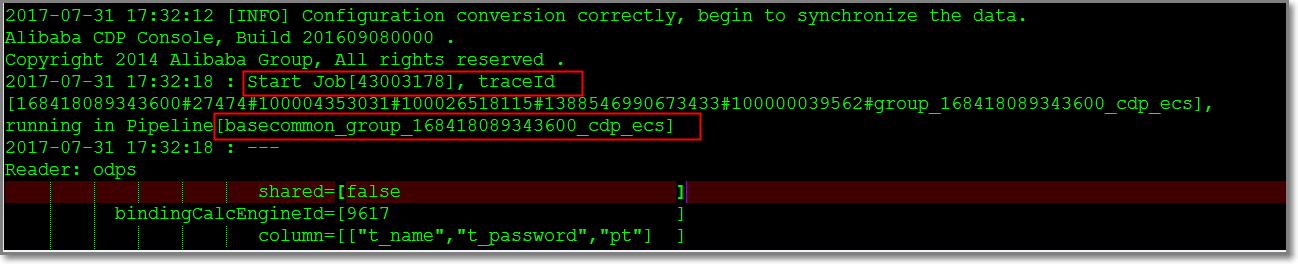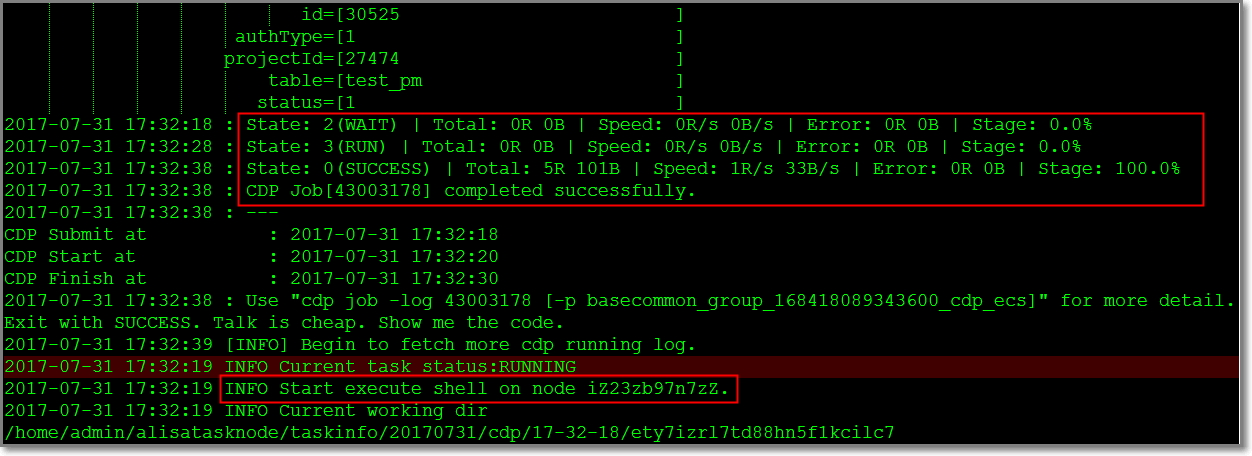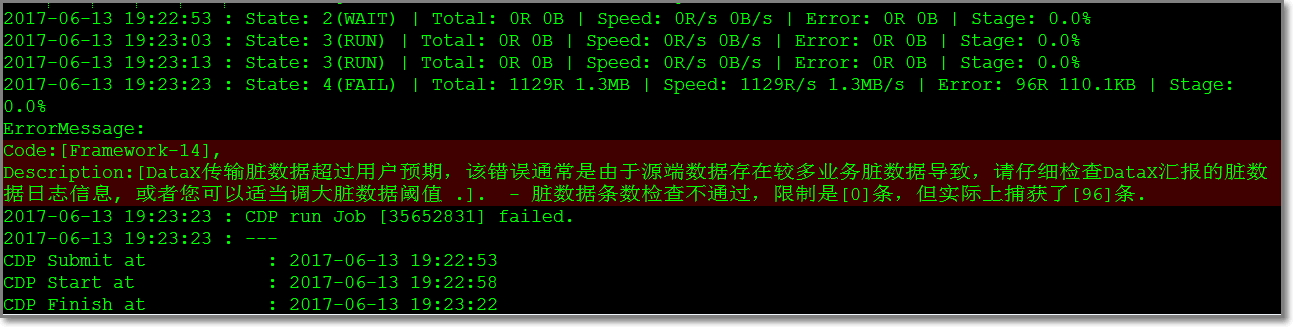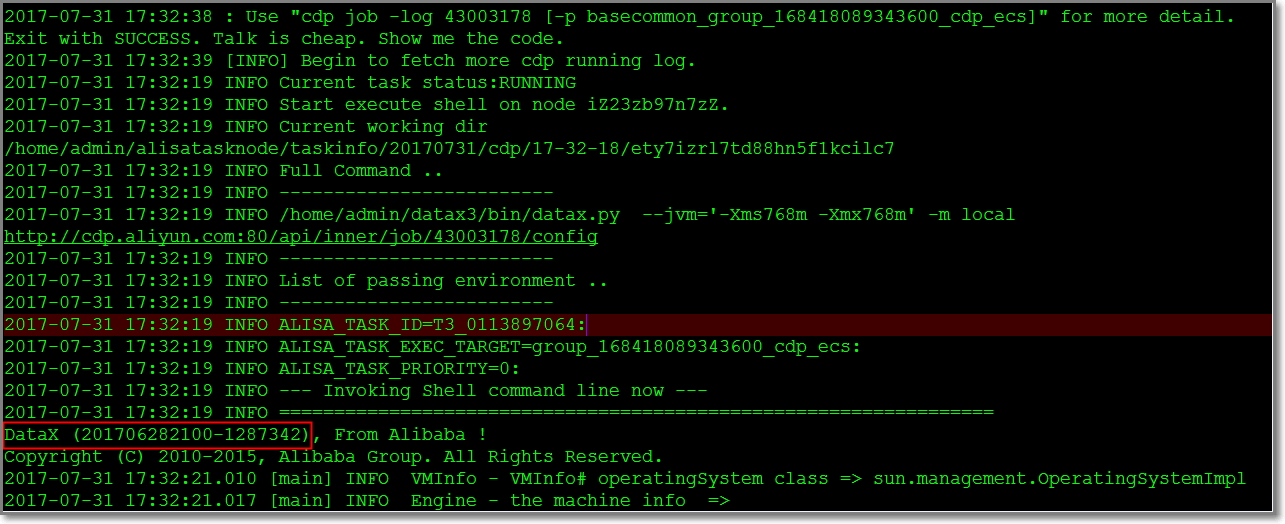# 步骤4：查看同步日志

### 任务是从哪里开始的### 实际运行的任务代码

``Reader: odps ``                       shared=[false                         ]``          bindingCalcEngineId=[9617                          ]``                       column=[["t_name","t_password","pt"]  ]``                  description=[connection from odps calc engine 9617]``                      project=[XXXXXXXXX                     ]``                   *accessKey=[********                      ]``                    gmtCreate=[2016-10-13 16:42:19           ]``                         type=[odps                          ]``                     accessId=[XXXXXXXXX                     ]``               datasourceType=[odps                          ]``                   odpsServer=[http://service.odps.aliyun.com/api]``                     endpoint=[http://service.odps.aliyun.com/api]``                    partition=[pt=20170425                   ]``             datasourceBackUp=[odps_first                    ]``                         name=[odps_first                    ]``                     tenantId=[168418089343600               ]``                      subType=[                              ]``                           id=[30525                         ]``                     authType=[1                             ]``                    projectId=[27474                         ]``                        table=[t_name                        ]``                       status=[1                             ]``Writer: odps ``                       shared=[false                         ]``          bindingCalcEngineId=[9617                          ]``                       column=[["id","name","pt"]            ]``                  description=[connection from odps calc engine 9617]``                      project=[XXXXXXXXX                     ]``                   *accessKey=[********                      ]``                    gmtCreate=[2016-10-13 16:42:19           ]``                         type=[odps                          ]``                     accessId=[XXXXXXXXX                     ]``               datasourceType=[odps                          ]``                   odpsServer=[http://service.odps.aliyun.com/api]``                     endpoint=[http://service.odps.aliyun.com/api]``                    partition=[                              ]``                     truncate=[true                          ]``             datasourceBackUp=[odps_first                    ]``                         name=[odps_first                    ]``                     tenantId=[XXXXXXXXX                     ]``                      subType=[                              ]``                           id=[30525                         ]``                     authType=[1                             ]``                    projectId=[27474                         ]``                        table=[test_pm                       ]``                       status=[1                             ]``

### 任务运行情况### 详细运行日志